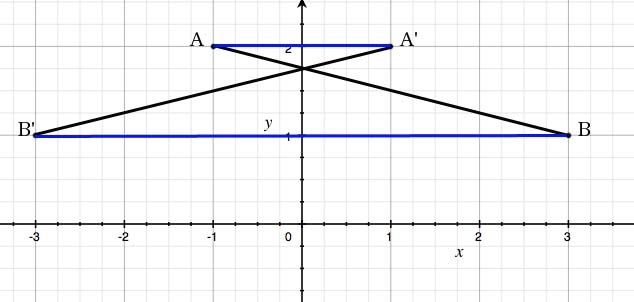SEARCH HOMEMath Central Quandaries & QueriesQuestion from Peter, a student: Segment AB has endpoints A(-1,2) and (3,1). Segment AB is reflected over the y-axis such that A becomes A' and B becomes B'. What is the positive difference between the lengths of segment AA' and segment BB'?Hi Peter,

The segment AB and its reflection A'B' looks like the following:Positive difference between the lengths means the same thing as the absolute value of the subtracted lengths. Since |AA'-BB'|=|A'A-BB'|, order does not matter.

JaniceMath Central is supported by the University of Regina and The Pacific Institute for the Mathematical Sciences.